• Call Now

1800-102-2727•

# Velocity-Time Graph: Acceleration Time, Practice Problems and FAQs

You get on your bike, start pedalling slowly, reaching a certain velocity. Since your velocity changed as a result of you pedalling intensively, surely you would have accelerated in the process. But how to find that acceleration if you have knowledge of time as well? One approach which comes to our mind is applying equations of motion; the other would be to plot a graph with velocity v on the vertical axis and time t on the horizontal axis; such a graph is called velocity-time graph or v-t graph. Similarly, a graph plotted with acceleration a on the vertical axis and time t on the horizontal axis is called acceleration time graph or a-t graph. Before we explore more about these graphs, it is vital to understand two important terms: slope and area. Slope is the tangent of the angle that a straight line or a line drawn to the curve makes with the x-axis; in the following figure for instance, the line drawn to the curve makes an angle of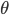with the +ve x-axis, then

slope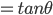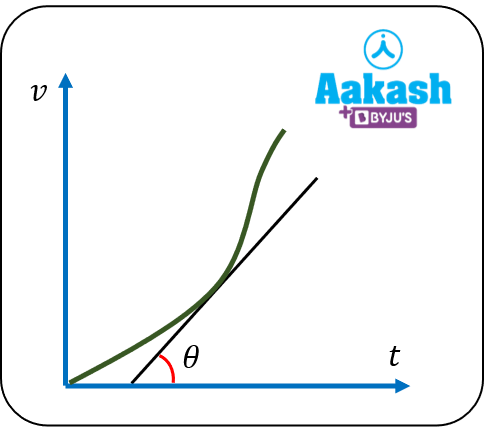Fig illustrating the slope of v-t graph

Area enclosed by the a-t graph with the t-axis gives the change in velocity.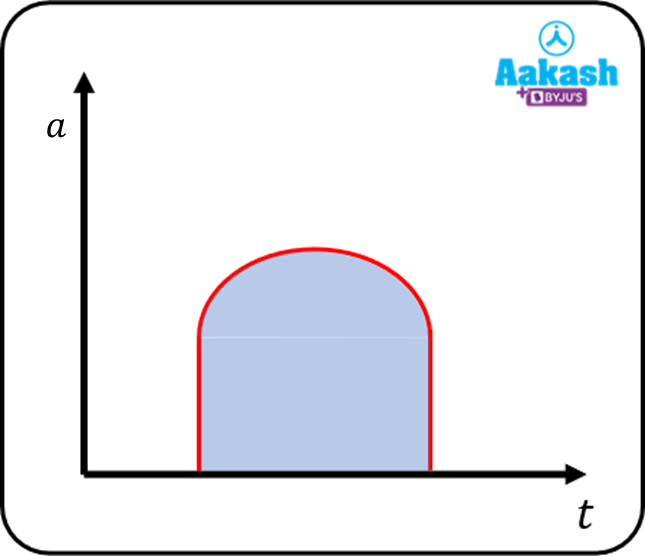Area under the a-t graph gives change in velocity

## Velocity Time Graph

• As the name itself suggests, a graph plotted between velocity v on the vertical axis and time t on the horizontal axis is called velocity time or v-t graph.
• Since acceleration a=dvdt; slope of the v-t graph gives acceleration. In the graphical sense, differentiating interprets to finding slope.
• x=vdt , area under the velocity time graph gives displacement (x)

In the following example, the slope of v-t graph in the interval 3-6 sec can be calculated as follows: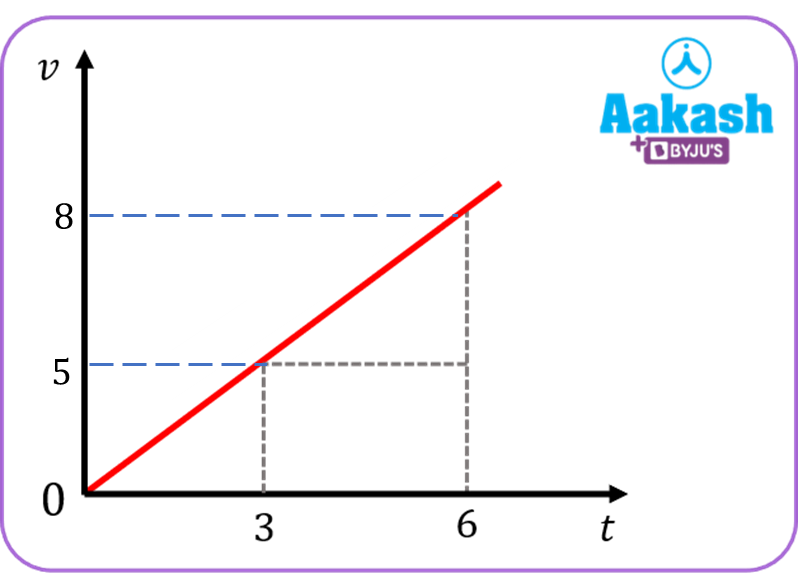• Area under the v-t graph gives the displacement. In the following figure, the displacement = area under the graph.

Displacement x = Area under the trapezium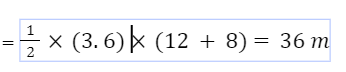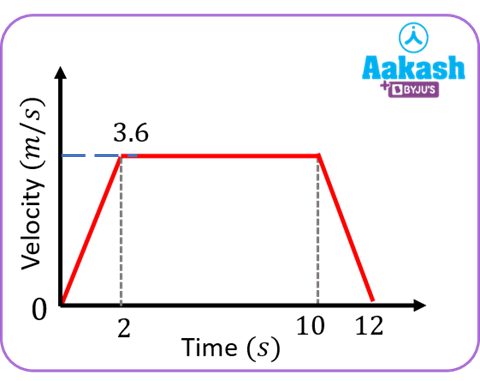## Acceleration time graph

• Graph plotted between acceleration on the y- axis and time t on the x-axis is called acceleration time or a-t graph.
• The area under a-t graph gives the change in velocity; since v=adt . Integration ,in the graphical sense, refers to the area under the curve.
• For a uniformly accelerated motion, acceleration is the same in all intervals of time .i.e it does not change. Hence the a-t graph for such a motion will be a straight line parallel to t-axis.Fig showing a-t graph for a uniformly accelerated motion

For a non uniform motion in which acceleration is not same, the a-t the graph could be as follows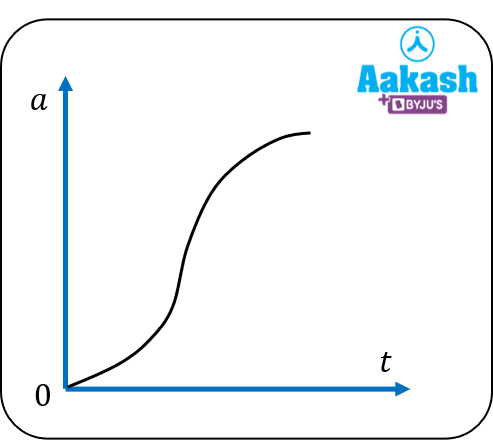Fig showing a-t graph for a non-uniformly accelerated motion

## Practice Problems of Velocity-Time Graph

Question.1 A ball is allowed to fall freely under gravity. Which of the following graphs represents its correct v-t graph?

(a)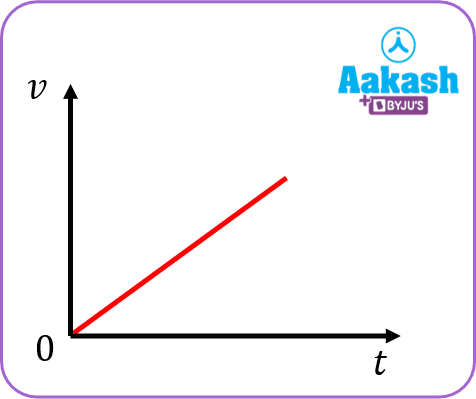(b)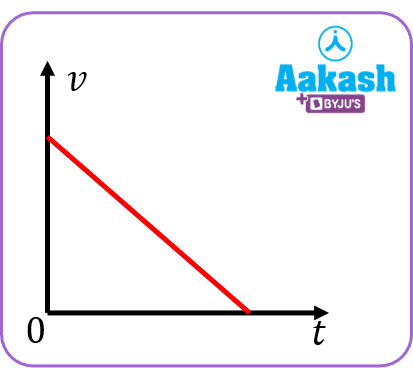(c)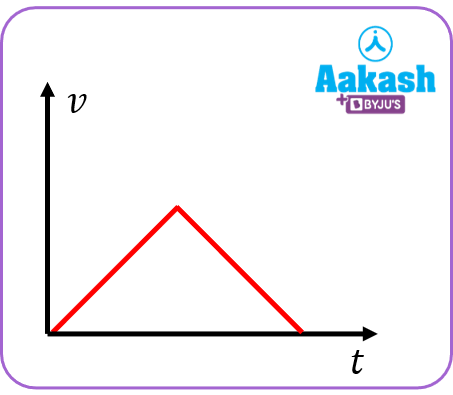(d)Answer: For a body falling freely under gravity, its acceleration is constant and speed keeps on increasing.

Question.2 Which of the following speed time graphs is not possible?

(a)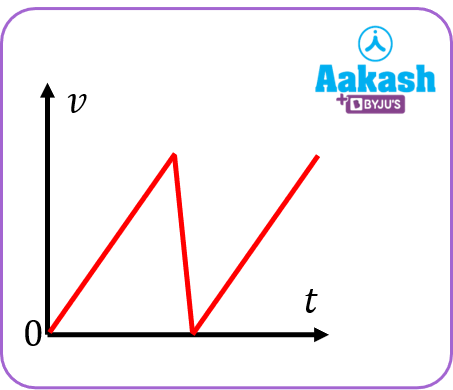(b)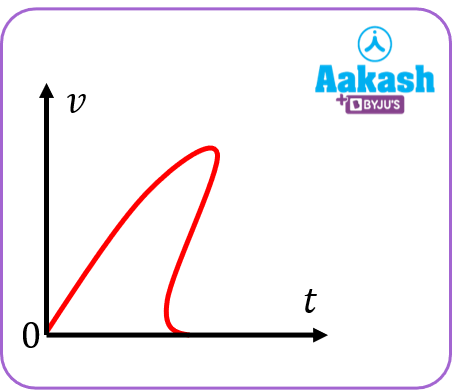(c)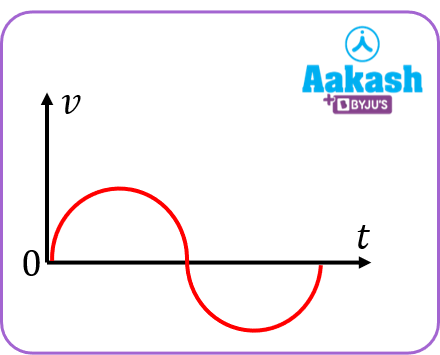(d) All of these

In (a) acceleration is not defined at cusp.

In (b) for the same value of time, different values of velocities are there.

In (c) speed becomes negative.

Hence (d) is the correct answer

Question.3 For the acceleration time graph shown in the figure; what is its corresponding v-t graph? Given that at t=0; v=0.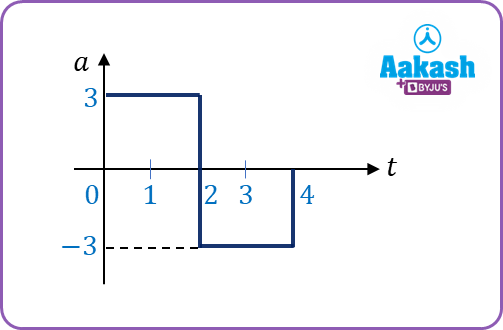(a)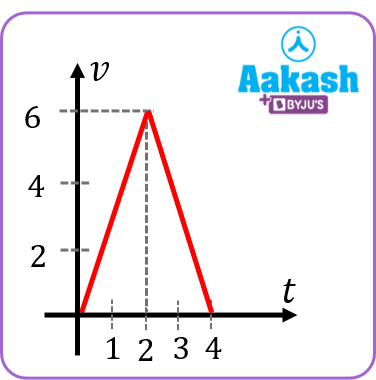(b)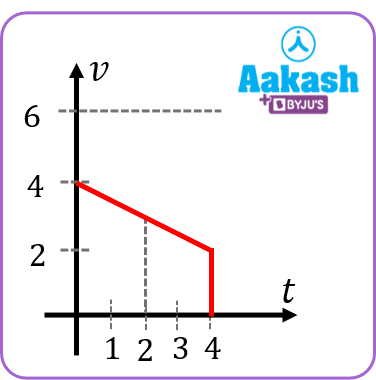(c)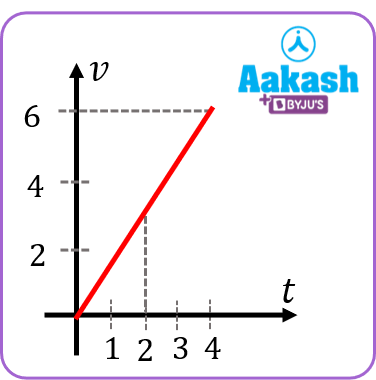(d) none of these

Answer: A Between t=0 & t=2;v=0+at;a=3 ms-2,t=2v=6 ms-1

Between t=2 & t=4;v=6+(-3)(2)v=0 ms-1

Question.4 The a-t graph of a particle moving along a straight line from rest is shown below. Which graph best represents its variation of velocity with time?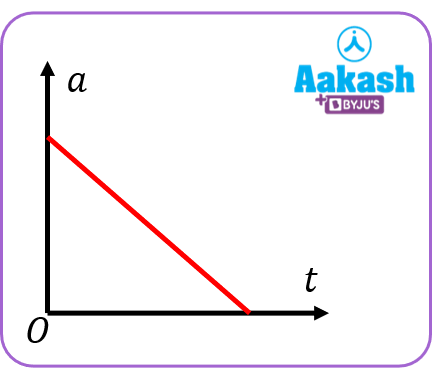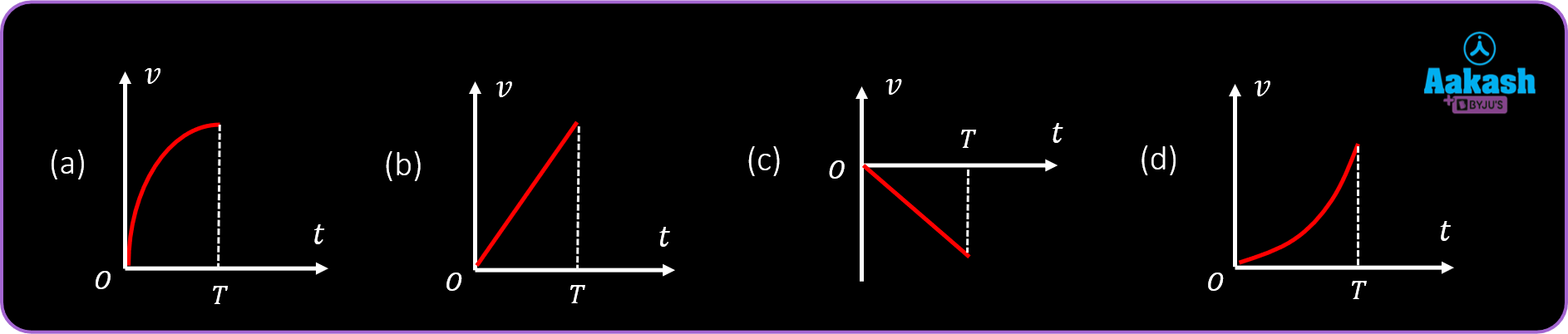The given graph can be written as a=-kt+c;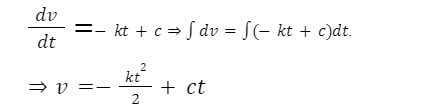This implies that the corresponding v-t graph is a parabola.

## FAQs of Velocity-Time Graph

Question.1 How to find acceleration from v-t graph?

Answer Slope of velocity time (v-t) graph gives acceleration.

Question.2 What is the v-t graph for a ball thrown upwards considering upwards as positive?

Answer: For a ball thrown upwards, acceleration a=-g. Hence slope of v-t graph is -ve.

Question.3 What does the area under the speed time graph give?

Answer: Area under the speed time graph gives distance.

Question.4 What does the velocity time graph look like for a non-uniform accelerated motion?

Answer: For a non-uniform accelerated motion, acceleration is not constant. Therefore the slope of v-t graph is not the same throughout; so a possible graph could be

## NCERT Class 11 Physics Chapters

 Physical World Units and Measurements Motion in a Straight Line Motion in a Plane Laws of Motion Work Energy and Power Particals and Rotational Motion Gravitation Mechanical Properties of Solids Mechanical Properties in Liquids Thermal Properties of Matter Thermodynamics Kinetic Theory Oscillations WavesTalk to our expert
Resend OTP Timer =
By submitting up, I agree to receive all the Whatsapp communication on my registered number and Aakash terms and conditions and privacy policy